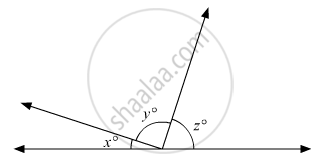# In the Given Figure, If Y X = 5 and Z X = 4 Then the Value of X Isc - Mathematics

MCQ

In the given figure, if $\frac{y}{x} = 5$ and $\frac{z}{x} = 4$ then the value of x isc•  8°

•  18°

•  12°

•  15°

#### Solution

In the given figure, we havex°,y° and z° forming a linear pair, therefore these must be supplementary.That is,

x + y +z = 180°                    (i)

Also,

y/x = 5

y = 5x          (ii)

And

z/x = 4

z = 4x         (iii)

Substituting (ii) and (iii) in (i), we get:

x + 5x + 4x = 180°

10x = 180°

x = (180°)/10

x = 18°

Concept: Parallel Lines and a Transversal
Is there an error in this question or solution?

#### APPEARS IN

RD Sharma Mathematics for Class 9
Chapter 10 Lines and Angles
Q 26 | Page 57